Home > CCA2 > Chapter 12 > Lesson 12.2.1 > Problem12-90

12-90.
1. Rewrite each of the following expressions in a simpler form. Homework Help ✎

1. cos2(θ π) + sin2(θ π)

2. cos2(2w) − sin2(2w)

3.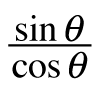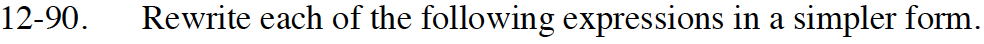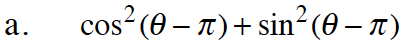Remember:
cos²(θ) + sin²(θ) = 1.
The variable here is different, but will this change the identity? If you aren't sure, let U = θ − π

1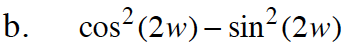Remember:
cos²(x) − sin²(x) = cos(2x).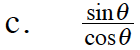Try writing sin(θ) as opposite over hypotenuse and cos(θ) as adjacent over hypotenuse.
Simplify the complex fraction. Is the new ratio equivalent to another trig ratio?

tan(θ)